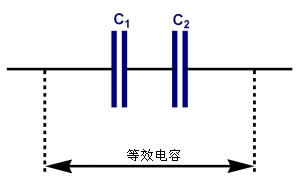# 电容串联的基本内容

## 电容串联的基本内容

2016-11-02 作者: xuzhiping 浏览: 2226 次$$C= \frac {C1C2}{C1+C2}$$

$$C= \frac {C1C2…Cn}{C1+C2+…+Cn}$$

1.总电荷持平$$Q=Q1=Q2$$

2.总电压等于各电容电压之和$$U=U1+U2$$

3.总容量倒数等于各电容倒数之和$$1/C=1/C1+1/C2$$

3.总电阻等于各电容电阻之和$$R=R1+R2$$